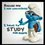# hyperbolic function proof 1

Eh guy, i decided to create this note to prove some hyperbolic identity...... Enjoy... A famous hyperbolic equation that we all know is: \cosh\^2{x} - \sinh\^2{x} =1 have you ever imagined how this was proved? lets take a look at it! bold$proof$

from hyperbolic identity, $\cosh{x}$= \frac{\exp\^x + \exp\^-x}{2} and $\sinh{x}$= \frac{\exp\^x - \exp\^-x}{2} lets add $\coshx$+ $\sinhx$ = \frac{\exp\^x + \exp\^-x}{2} + \frac{\exp\^x - \exp\^-x}{2} using method of L.C.M, = \frac{2$$exp\^x ) = bold \exp\^x now lets subtract, $\coshx$- $\sinhx$ = \frac{\exp\^x + \exp\^-x}{2} - \frac{\exp\^x - \exp\^-x}{2} = \frac{2\( exp\^-x ) = bold \exp\^-x then lets multiplty =$\coshx$+ $\sinhx$ * $\coshx$- $\sinhx$ = \exp\^x * \exp\^-x = \exp\^x * \frac{1}{\exp\^x} =bold$1$ so guys, i hope you enjoyed this? like and share! :)Note by Samuel Ayinde 7 years, 1 month ago This discussion board is a place to discuss our Daily Challenges and the math and science related to those challenges. Explanations are more than just a solution — they should explain the steps and thinking strategies that you used to obtain the solution. Comments should further the discussion of math and science. When posting on Brilliant: • Use the emojis to react to an explanation, whether you're congratulating a job well done , or just really confused . • Ask specific questions about the challenge or the steps in somebody's explanation. Well-posed questions can add a lot to the discussion, but posting "I don't understand!" doesn't help anyone. • Try to contribute something new to the discussion, whether it is an extension, generalization or other idea related to the challenge. • Stay on topic — we're all here to learn more about math and science, not to hear about your favorite get-rich-quick scheme or current world events. MarkdownAppears as *italics* or _italics_ italics **bold** or __bold__ bold - bulleted- list • bulleted • list 1. numbered2. list 1. numbered 2. list Note: you must add a full line of space before and after lists for them to show up correctly paragraph 1paragraph 2 paragraph 1 paragraph 2 [example link](https://brilliant.org)example link > This is a quote This is a quote  # I indented these lines # 4 spaces, and now they show # up as a code block. print "hello world" # I indented these lines # 4 spaces, and now they show # up as a code block. print "hello world" MathAppears as Remember to wrap math in \( ... $$ or $ ... $ to ensure proper formatting.
2 \times 3 $2 \times 3$
2^{34} $2^{34}$
a_{i-1} $a_{i-1}$
\frac{2}{3} $\frac{2}{3}$
\sqrt{2} $\sqrt{2}$
\sum_{i=1}^3 $\sum_{i=1}^3$
\sin \theta $\sin \theta$
\boxed{123} $\boxed{123}$

## Comments

There are no comments in this discussion.

×

Problem Loading...

Note Loading...

Set Loading...